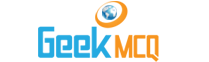# Entry Test MCQ :: Logarithm

@ : Home > Math > Logarithm

1.  If log10 36 = y what does log10 36000 equal?
 A. y B. y+1000 C. 1000 D. y+3 E. y+1

2.  If log10 2 = x and log10 3 = y find the value of log10 80?
 A. y+1 B. x+y C. x-y D. x+2y E. none

3.  Solve for a : log (a+3) = loga+log3
 A. 1 B. 3?2 C. 2?3 D. 6?5 E. none

4.  Evaluate log16 ?56 - log16 ?14
 A. 0 B. 1?4 C. 1 D. 3 E. 1?3

5.  Evaluate log9 3 - log5 125 + log625 5 - log7 (343)1/3
 A. 0 B. 1?3 C. 3?28 D. ?13?4 E. none

6.  If Log10 G= 1/5 then log10 100G5=?
 A. 7 B. 1?4 C. 4 D. 11?4 E. 3

7.  If Logy 6=x logy 3.5 = z and 6a = 3.5 then a = __________
 A. xz B. z/x C. x+z D. z-x E. not determined

8.  If g(x) = log2 y then g(3y) - g(y) = ___________
 A. log(2/x)+log2x B. log2 (2+x2)/x C. 1 D. log23 E. log3-log2

9.  If log5 p = ?8 and log 8 q = ?5 then pq = _________
 A. 5?8.8?5 B. 13?13 C. 3?3 D. 7?2 E. 0

10.  If log64 7 = x log4 7 then x = __________
 A. 4 B. log43 C. 3 D. 1?4 E. log464